Next: 4.8.2 An In-Segregation Model Up: 4.8 Examples Previous: 4.8 Examples

## 4.8.1 A Pair-Diffusion Model in One Dimension

A five species phosphorus diffusion model introduced by Richardson and Mulvaney [Ric92] has been developed. The species behavior can be formulated by the following system of coupled reaction diffusion equations= DVV - kEfor P V + kErev E - kbi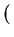V I - Veq Ieq(4.13)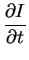= DII - kEfor P I + kErev F - kbiV I - Veq Ieq(4.14)= - kEfor P V + kErev E - kFfor P I + kFrev F (4.15)= DEE + kEfor P V - kErev E (4.16)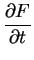= DFF + kFfor P I - kErev F (4.17)

where the solution vector u =V, I, P, E, F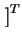represents, respectively, the concentration of vacancies, interstitials, substitutional phosphorus as well as phosphorus-vacancy pairs (E-centers) and phosphorus-interstitial pairs (F-centers).

Furthermore, zero flux boundary conditions for all species are enforced everywhere except at the exposed surfaces where V, I, P, E, and F are specified with

V = Veq  and I = Ieq
P = C*  (ambient gas concentration)
E =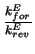P V
F =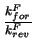P I

Using a finite element discretization within the analytical model interface the complete formulation of the discretized differential equation system is written in the code segment as shown below. The predefined variables like X,Y,Z (coordinates of the element points) and t (time) are initialized during runtime and can be used like any other variable within the model definition language.

MODEL PairDiff = [V,I,E,F,P];
{

############## Discretization ################################

N(xsi) = [1-xsi,xsi];        # the element shape function defined as
# a function of xsi
dNdxsi = [ -1  , 1 ];        # derivative of the shape function

dxdxsi = dNdxsi * X;         # X is a predefined vector [X1,X2,...,Xn]
# getting real size and coordinates
# during runtime

detJ = |dxdxsi|;
dxsidx = Inv(dxdxsi);        # calculates the inverse matrix

L = dNdxsi*dxsidx;           # gradient operator
K = L^T*L;                   # Laplace operator where (^T) is a
# transpose operator
C = N(1/2)*N(1/2)^T;         # time operator using lumping
# N(1/2) -> calculates the value
#           of the shape function
#           for xsi=0.5

############## The Parameters #################################

Param k_for_e = 1.0E-14;     # in cm^3/s
Param k_for_f = 1.0E-14;     # initializing default values
Param k_bi = 1.0E-10;        # for parameters

Param k_rev_e = 10;          # in 1/s
Param k_rev_f = 12;

Param Dv  = 1.0E-10;         # in cm^2/s
Param Di  = 1.0E-09;
Param De  = 1.0E-13;
Param Df  = 2.0E-13;

Param Veq  = 1.0E14;         # in 1/cm^3
Param Ieq = 1.0E14;

############## The Differential Equations #####################

dV = V.t0-V.t1;              # of unknown and predefined
dI = I.t0-I.t1;              # variables
dE = E.t0-E.t1;
dF = F.t0-F.t1;
dP = P.t0-P.t1;

i = 1..2;                    # running variable from 1 to 2

PV[i]  = P[i] * V[i];
PI[i]  = P[i] * I[i];
VI[i]  = I[i] * V[i];
VIeq[i] = Veq * Ieq;

resV = detJ * (Dv*K*V + C * (dV/dt-k_for_e*PV+k_rev_e*E-k_bi*(VI-VIeq)));
resI = detJ * (Di*K*I + C * (dI/dt-k_for_f*PI+k_rev_f*F-k_bi*(VI-VIeq)));
resE = detJ * (De*K*E + C * (dE/dt+k_for_e*PV-k_rev_e*E));
resF = detJ * (Df*K*F + C * (dF/dt+k_for_f*PI-k_rev_f*F));
resP = detJ *           C * (dP/dt-k_for_e*PV+k_rev_e*E-k_for_f*PI+k_rev_f*F);

############## The Residual and its Derivative #####################

residuum = [[resV][resI][resE][resF][resP]];     # the residual vector
jacobian = D([[V][I][E][F][P]],residuum^T)^T;    # auto derivative
# of residual
}


To define the necessary simulation parameters and to map the developed analytical pair-diffusion model onto a definite simulation domain including its boundaries the specifications of the Input & Control Interface (Section 4.2) have to look like:

# Where to read the grid from
Source=Pif
{
Physical = lin2.pbf    # the input filename
Logical  = BareWafer   # the logical name of the mesh
}
# Where to write the grid and the result to
Output = Pif
{
Physical = lin2res.pbf # the output filename
Logical  = BareWafer   # the logical name of the new generated
# output mesh
}

Time = 60                  # the first 60 seconds
{
Step    = 1.0E-3        # initial timestep
Epsilon = 1.0E-3        # maximum error
RejectionRatio = 0.5    # reduce step-size in case of non
# converging or epsilon error
StretchRatio   = 1.5    # increase step-size in case of success
MaxNewton      = 15     # stop after 15 iterations and reduce step
# size
}

Grid = Si_Region  # the name of the grid in the default input file
{
Model = PairDiff(V,I,E,F,P)   # use the model PairDiff
{                             # and map the quantities
Value(V) = 1.0E14         # initialize the quantity V
Value(I) = 1.0E14         # initialize the quantity I
Value(E) = 0.0E0          # initialize the quantity E
Value(F) = 0.0E0          # initialize the quantity F
Value(P) = 0.0E0          # initialize the quantity P
}
}

Boundary = Backside         # any name because auto boundary detection
# is used
{
Interface(Si_Region,Top) # Choose the top boundary of the grid with
# name Si_Region

# Use Dirichlet boundaries for the defined quantities

Model = Dirichlet(V){Value(V) = 1.0E14}
Model = Dirichlet(I){Value(I) = 1.0E14}
Model = Dirichlet(P){Value(P) = 5.0E20}
Model = Dirichlet(E){Value(E) = 5.0E19}
Model = Dirichlet(F){Value(F) = 4.16666E19}
}


Finally the simulation results that show the distribution of all five species after 60 seconds (Fig. 4.14) are written to the file defined in the output section. Fig. 4.15 shows the result for the same model calculating the distribution after 600 seconds.Next: 4.8.2 An In-Segregation Model Up: 4.8 Examples Previous: 4.8 Examples